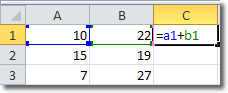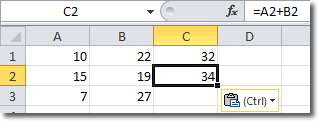# Planet For Application Life Development Presents MY IT WorldExplore and uptodate your technology skills...

Excel - Absolute Reference

By default, cell references in Excel are relative. For example, if you have the following formula in cell C1:and you copy the formula to cell C2, you will get =A2+B2. This is because the cell references are relative. The contents of cell C1 are calculated to be the sum of the the cell that is 2 cells to the left and the cell that is 1 cell to the left. This easily transposes to cell C2.

To demonstrate, I copied cell C1 to cell C2 and got this:You can see in the formula bar that the original formula of =A1+B2 has been transformed to =A2+B2 when copied to cell C2.

But what if we want to always use A1+B1 in all cells we copy that formula to? This is where absolute cell references come in.

## How To Construct An Absolute Cell Reference

In Excel, we create an absolute cell reference by using a “\$” before each letter and number. The formula for C1 then becomes

=\$A\$1+\$B\$2

Copying that formula to C2 retains those absolute references and we find the that C2 also uses =\$A\$1+\$B\$2.

You can also mix ansolute with relative references like this: A\$1. Here the row is fixed at one, but the column remains relative.